#### Likelihood Function

Let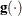be a link function such that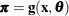whereis a column vector for regression coefficients. The pseudo-log likelihood is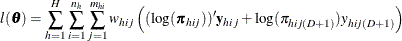Denote the pseudo-estimator as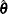, which is a solution to the estimating equations: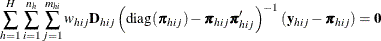where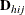is the matrix of partial derivatives of the link function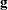with respect to.

To obtain the pseudo-estimator, the procedure uses iterations with a starting value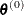for. See the section Iterative Algorithms for Model Fitting for more details.